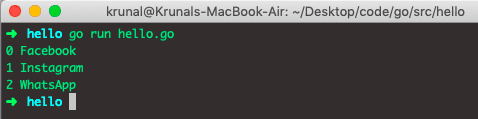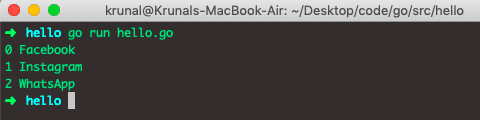# Golang For Loop: How to Iterate Variables in Go

Golang doesn’t have a while, or do-while loops, which are present in other languages like C. But Golang has for loop. Now let’s go back to the history of iteration.

## Survey of Definite Iteration in Programming

Definite iteration loops are usually referred to as for loops because they are the keyword used to propose them in nearly all programming languages, including Golang. Historically, all the languages have offered a few assorted flavors of for loop.

### Numeric Range Loop

The most fundamental for loop is a simple numeric range statement with start and end values. The exact format differs depending on the language but typically looks something like the following syntax.

```for k = 1 to 10
<loop body>```

Here, the body of a loop is executed ten times. The variable k assumes the value 1 on the first iteration, 2 on the second, etc. This kind of for loop is used in languages Algol, BASIC, and Pascal.

### Three-Expression Loop

Another form of for loop generalized by the C language contains three parts:

1. An initialization
2. An expression specifying an ending condition
3. The operation is to be performed at the end of each iteration.

This type of loop has the following format.

```for (k = 1; k <= 10; k++)
<loop body>```

The for loop is interpreted as follows:

1. Initialize k to 1.
2. Continue looping as long as k<=10.
3. Increment k by 1 after each loop iteration.

Three-expression for loops is famous because the expressions specified for three parts can be nearly anything, so this has more extensibility than the simpler numeric range form shown above. These for loops are also recommended in C++, PHP, Java, and Perl languages.

### Collection-Based or Iterator-Based Loop

As the name suggests, the collection-based loop iterates over a collection of objects rather than specifying numeric values or conditions.

```for i in <collection>
<loop body>```

Each time through the loop, the variable k takes on the value of the next object in <collection>. This kind of for loop is arguably the most generalized and abstract. PHP and Perl also support this kind of loop, but it is introduced by the keyword foreach instead of for.

## Golang for loop

Golang for loop is a built-in statement used to execute a block of code repeatedly. Go for loop supports three expressions, and semicolons separate it:

1. The init statement: The init statement is executed before the first iteration. This step allows us to declare and initialize any for loop control variables. You are not required to put the statement here as long as the semicolon appears.
2. The condition expression: This statement is evaluated before every iteration. If a condition is true, the body of the loop is executed. If the condition is false, the loop’s body does not execute, and the control flow jumps to the next statement just after a for loop.
3. The post statement: This statement is executed at the end of every iteration. If the range is available, then the for loop executes for each item in the range.

The for loop in Golang without a condition will repeatedly loop until you break out of the loop or return from the enclosing function. You can also continue to the next iteration of the loop.

The syntax for loop in Golang is the following.

### Syntax

```for [condition |  ( init; condition; increment ) | Range] {
body
}```

### Go for loop example

Let’s see the example.

```/* hello.go */

package main

func main() {

for i := 0; i < len(apps); i++ {
println(i, apps[i])
}
}
```

Okay, so we have defined one slice called apps. Then we iterated that variable in for loop and got the following output.The for loop will stop iterating once the boolean condition evaluates to false.

In this example, the iterable is the slice apps, and i is the variable. Each time through a loop, i takes on the successive item in a, so print() displays the values’ Facebook’, ‘Instagram’, and ‘Whatsapp’, respectively. A for loop like this is the Go way to process the items in an iterable.

Unlike the other languages like C, C++, Java, or JavaScript, no parentheses are required to surround the three components of a for statement, but the braces { } are always required.

## For…range() in Golang

In the first section of this example, you saw a type of for loop called the numeric range loop, in which starting and ending numeric values are specified. Although this form of for loop isn’t directly built into Golang, it is quickly arrived at.

### Golang range()

The range form of the for loop iterates over a slice or map. When ranging over a slice, two values are returned for each iteration. The first is the index, and the second is a copy of the element at that index.

Thus, the range object is iterable, and you can obtain the values by iterating over them with a for loop.

Let’s see the following example.

```/* hello */

package main

import "fmt"

func main() {

for i, app := range apps {
fmt.Println(i, app)
}
}
```

The output of the above code is the same.We have used the range keyword when we need to iterate each element of the slice in Go.

With an array and slices, it returns an index of the element as an integer. With maps, it returns the key of the next key-value pair.

The range() method either returns one value or two. If only one value is used on the left of the range expression, it is the first value in the following table.

Range expression First Value Second Value(Optional)
Array or slice an [n]E index i int a[i] E
String s string type index i int rune int
map m map[K]V key k K value m[k] V
channel c chan E element e E none

## Conclusion

In this example, we have seen the type of for loops, including Numeric loop, three expressions loop, collection-based loop, Golang for loop, and Golang for loop with range() function.

That’s it for this tutorial.

Golang Constant

Golang Map

Golang Array

Golang Struct